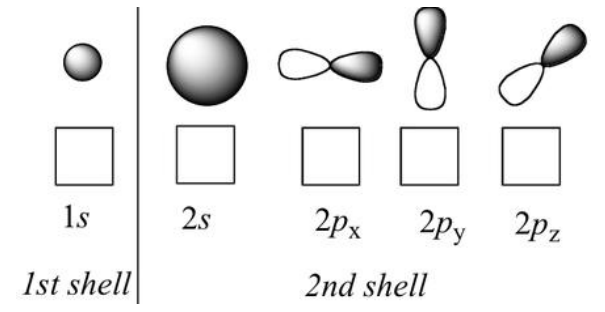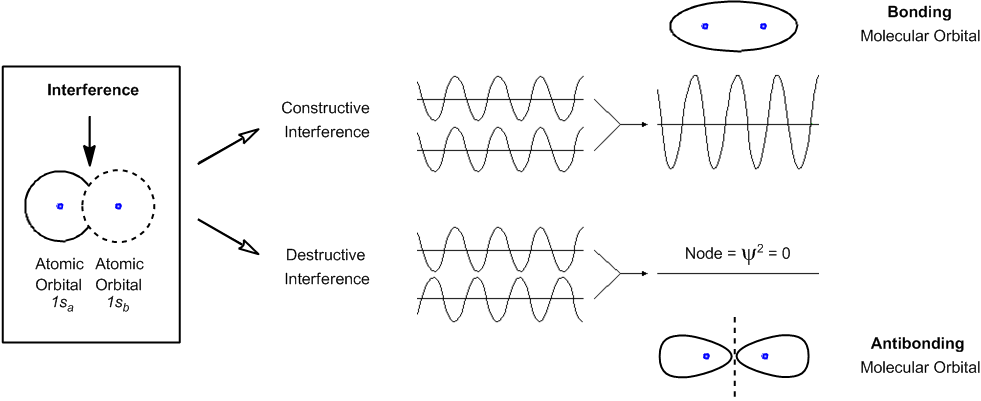Start typing, then use the up and down arrows to select an option from the list.# Organic Chemistry

Learn the toughest concepts covered in Organic Chemistry with step-by-step video tutorials and practice problems by world-class tutors.

1. A Review of General Chemistry

This section deals with the basics of quantum mechanics. Don’t worry too much about it, but this is some good general information.

Definition of an Atomic Orbital:These funny orbital shapes represent the 3-D plots of the equations that describe the probability of finding electrons at any given place as their energy states increase.

1

#### concept

The probability of finding electrons in a given place.6m
Play a video:

## Atomic Orbital Interference

Instead of colliding into each other, wave functions have the ability to interfere with each other upon meeting.The type of interference determines if a new bond will be created between the two orbitals.

2

#### concept

Constructive vs. destructive interference.3m
Play a video: